Next: Constrained Lagrangian Dynamics Up: Hamiltonian Dynamics Previous: Multi-Function Variation

Hamilton's Principle

We saw, in Chapter 9, that we can specify the instantaneous configuration of a conservative dynamical system with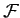degrees of freedom in terms ofindependent generalized coordinates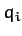, for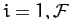. Let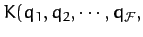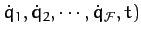and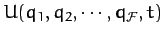represent the kinetic and potential energies of the system, respectively, expressed in terms of these generalized coordinates. Here,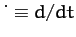. The Lagrangian of the system is defined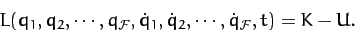(711)

Finally, theLagrangian equations of motion of the system take the form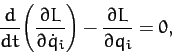(712)

for.

Note that the above equations of motion have exactly the same mathematical form as the Euler-Lagrange equations (708). Indeed, it is clear, from Section 10.4, that theLagrangian equations of motion (712) can all be derived from a single equation: namely,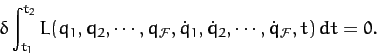(713)

In other words, the motion of the system in a given time interval is such as to maximize or minimize the time integral of the Lagrangian, which is known as the action integral. Thus, the laws of Newtonian dynamics can be summarized in a single statement:
The motion of a dynamical system in a given time interval is such as to maximize or minimize the action integral.
(In practice, the action integral is almost always minimized.) This statement is known as Hamilton's principle, and was first formulated in 1834 by the Irish mathematician William Hamilton.Next: Constrained Lagrangian Dynamics Up: Hamiltonian Dynamics Previous: Multi-Function Variation
Richard Fitzpatrick 2011-03-31